# Let's solve the simultaneous equations with Excel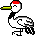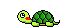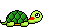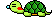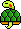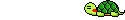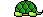Recommendation of matrix

 Let's think about the following 3-D simultaneous equations. 　（The variable is x1, x2, x3) 　　　5x1 + 3x2 + x3 = 3 　　　4x1 + 5x2 + 2x3 = 4 　　　　x1 + 3x2 + 6x3 = 6 When I transcribe it in a matrix.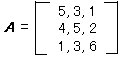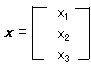It is extremely easy when written by a matrix 　　A　×x = b

Solution of simultaneous equations

 The simultaneous equations can be written by the following matrix equation 　　Ax = b Do multiplication a inverse-matrixA-1 in both sides. 　　　A-1( A x ) = A-1b 　　　(A-1A ) x = A-1b 　　From A-1A = E 　　　E x = A-1b 　　　x = A-1b Solution x calculates in this.

The simultaneous equations is solved with EXCEL

(1) Input of matrix (A) and vector (b)

 The matrix and the vector area are secured for an arbitrary cell, and the value is input.(2) Calculation of inverse-matrix ( A-1 )

 Select the inverse-matrixA-1 erea in an arbitrary position. . And set the Function MINVERSE (matrix A) . Note)  When you set the function to two or more areas  The OK button is not pushed. Enter is ended by the key input while pushing the Ctrl Shift key.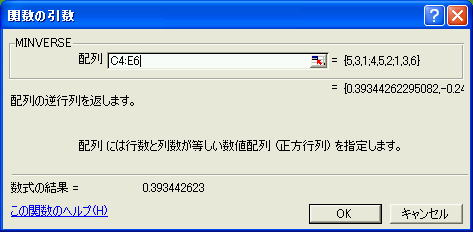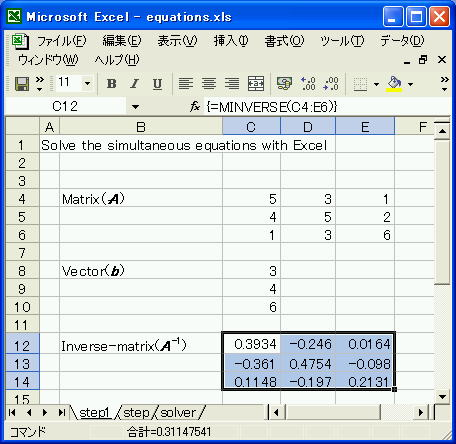The inverse-matrix calculated in this.

(3)Calculation of inverse-matrix(A-1) ×vector (b)

 Set a solution(x) earea in an arbitrary position. When you set the Function  MMULT(  Reverse-matrix(A-1), Vector(b))Solution  ( x ) However, it is round.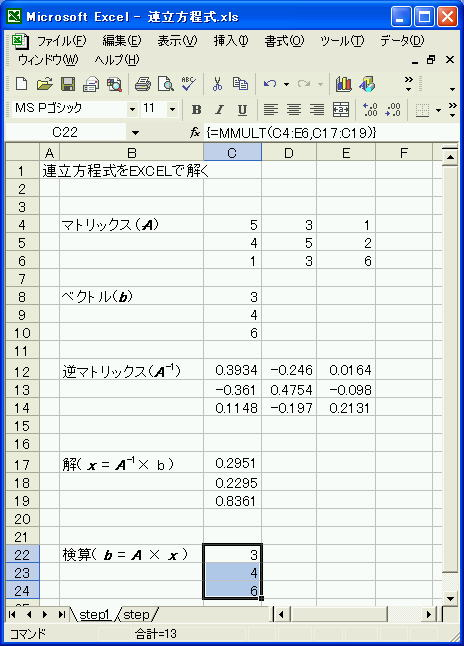the solution of the simultaneous equations was obtained. It is easy

 Obtained solution (x) is used.   Ax  Calculation, and the value agreement with b.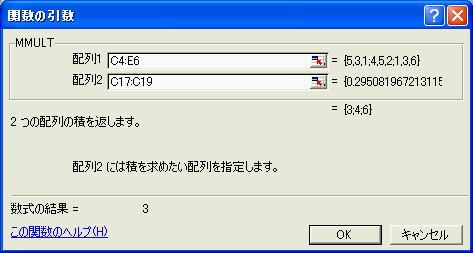Result of checking answer and vector b are corresponding. It is happy, and happy.Science and technology calculation with EXCEL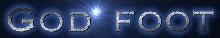〒673-0036  Japan Hyogo Prefecture Akeshi City Matsue 62-14
God foot co Ltd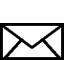Please let me hear the impression